# sbioelementaryeffects

Perform global sensitivity analysis (GSA) by computing elementary effects (requires Statistics and Machine Learning Toolbox)

Since R2021b

## Syntax

``elementaryEffectsResults = sbioelementaryeffects(modelObj,params,observables)``
``elementaryEffectsResults = sbioelementaryeffects(modelObj,params,observables,Name=Value)``

## Description

example

````elementaryEffectsResults = sbioelementaryeffects(modelObj,params,observables)` performs a global sensitivity analysis of a SimBiology model `modelObj` by computing elementary effects of `observables` with respect to individual model quantities or parameters specified in `params`.```

example

````elementaryEffectsResults = sbioelementaryeffects(modelObj,params,observables,Name=Value)` uses additional options specified by one or more name-value arguments.```

## Examples

collapse all

`sbioloadproject tumor_growth_vpop_sa.sbproj`

Get a variant with estimated parameters and the dose to apply to the model.

```v = getvariant(m1); d = getdose(m1,'interval_dose');```

Get the active configset and set the tumor weight as the response.

```cs = getconfigset(m1); cs.RuntimeOptions.StatesToLog = 'tumor_weight';```

Simulate the model and plot the tumor growth profile.

`sbioplot(sbiosimulate(m1,cs,v,d));`Perform global sensitivity analysis (GSA) on the model to find the model parameters that the tumor growth is sensitive to.

First, define model parameters of interest, which are involved in the pharmacodynamics of the tumor growth. Define the model response as the tumor weight.

```modelParamNames = {'L0','L1','w0','k1'}; outputName = 'tumor_weight';```

Then perform GSA by computing the elementary effects using `sbioelementaryeffects`. Use `100` as the number of samples and set `ShowWaitBar` to `true` to show the simulation progress.

```rng('default'); eeResults = sbioelementaryeffects(m1,modelParamNames,outputName,Variants=v,Doses=d,NumberSamples=100,ShowWaitbar=true);```

Show the median model response, the simulation results, and a shaded region covering 90% of the simulation results.

`plotData(eeResults,ShowMedian=true,ShowMean=false);`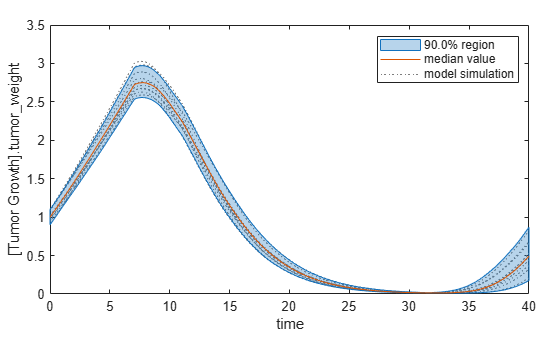You can adjust the quantile region to a different percentage by specifying `Alphas `for the lower and upper quantiles of all model responses. For instance, an `alpha` value of 0.1 plots a shaded region between the `100*alpha` and `100*(1-alpha)` quantiles of all simulated model responses.

`plotData(eeResults,Alphas=0.1,ShowMedian=true,ShowMean=false);`Plot the time course of the means and standard deviations of the elementary effects.

```h = plot(eeResults); % Resize the figure. h.Position(:) = [100 100 1280 800];```The mean of effects explains whether variations in input parameter values have any effect on the tumor weight response. The standard deviation of effects explains whether the sensitivity change is dependent on the location in the parameter domain.

From the mean of effects plots, parameters `L1` and `w0` seem to be the most sensitive parameters to the tumor weight before the dose is applied at t = 7. But, after the dose is applied, `k1` and `L0` become more sensitive parameters and contribute most to the after-dosing stage of the tumor weight. The plots of standard deviation of effects show more deviations for the larger parameter values in the later stage (t > 35) than for the before-dose stage of the tumor growth.

You can also display the magnitudes of the sensitivities in a bar plot. Each color shading represents a histogram representing values at different times. Darker colors mean that those values occur more often over the whole time course.

`bar(eeResults);`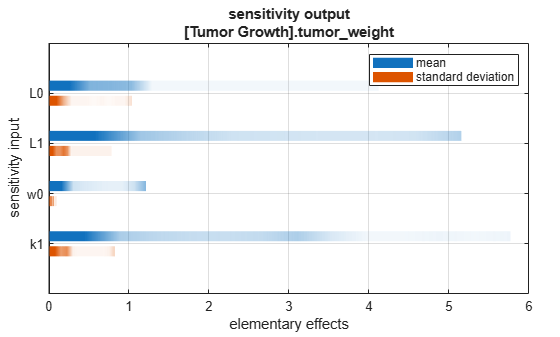You can also plot the parameter grids and samples used to compute the elementary effects.

`plotGrid(eeResults)`You can specify more samples to increase the accuracy of the elementary effects, but the simulation can take longer to finish. Use `addsamples` to add more samples.

`eeResults2 = addsamples(eeResults,200);`

The `SimulationInfo` property of the result object contains various information for computing the elementary effects. For instance, the model simulation data (SimData) for each simulation using a set of parameter samples is stored in the `SimData` field of the property. This field is an array of `SimData` objects.

`eeResults2.SimulationInfo.SimData`
``` SimBiology SimData Array : 1500-by-1 Index: Name: ModelName: DataCount: 1 - Tumor Growth Model 1 2 - Tumor Growth Model 1 3 - Tumor Growth Model 1 ... 1500 - Tumor Growth Model 1 ```

You can find out if any model simulation failed during the computation by checking the `ValidSample` field of `SimulationInfo`. In this example, the field shows no failed simulation runs.

`all(eeResults2.SimulationInfo.ValidSample)`
```ans = logical 1 ```

You can add custom expressions as observables and compute the elementary effects of the added observables. For example, you can compute the effects for the maximum tumor weight by defining a custom expression as follows.

```% Suppress an information warning that is issued. warnSettings = warning('off', 'SimBiology:sbservices:SB_DIMANALYSISNOTDONE_MATLABFCN_UCON'); % Add the observable expression. eeObs = addobservable(eeResults2,'Maximum tumor_weight','max(tumor_weight)','Units','gram');```

Plot the computed simulation results showing the 90% quantile region.

```h2 = plotData(eeObs,ShowMedian=true,ShowMean=false); h2.Position(:) = [100 100 1500 800];```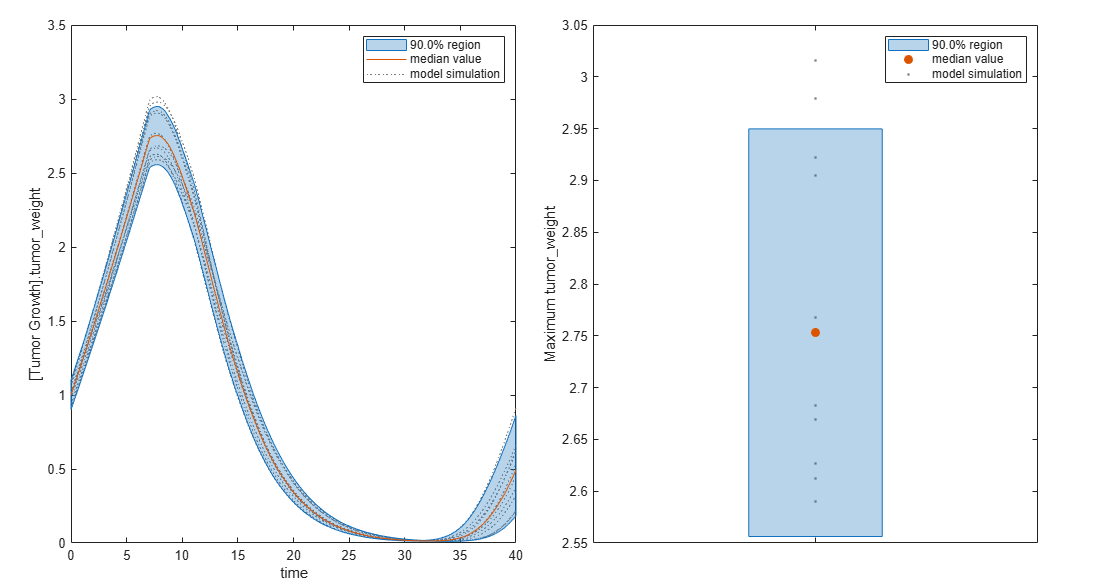You can also remove the observable by specifying its name.

`eeNoObs = removeobservable(eeObs,'Maximum tumor_weight');`

Restore the warning settings.

`warning(warnSettings);`

## Input Arguments

collapse all

SimBiology model, specified as a SimBiology `model object`.

Names of model parameters, species, or compartments, specified as a character vector, string, string vector, or cell array of character vectors.

Example: `["k1","k2"]`

Data Types: `char` | `string` | `cell`

Model responses, specified as a character vector, string, string vector, or cell array of character vectors. Specify the names of species, parameters, compartments, or `observables`.

Example: `"tumor_growth"`

Data Types: `char` | `string` | `cell`

### Name-Value Arguments

Specify optional pairs of arguments as `Name1=Value1,...,NameN=ValueN`, where `Name` is the argument name and `Value` is the corresponding value. Name-value arguments must appear after other arguments, but the order of the pairs does not matter.

Example: ```eeResults = sbioelementaryeffects(modelObj,params,observables,StopTime=10)``` specifies to use a stop time of 10.

Parameter bounds, specified as a numeric matrix with two columns. The first column contains the lower bounds and the second column contains the upper bounds. The number of rows must be equal to the number of parameters in `params`.

If a parameter has a nonzero value, the default bounds are ±10% of the value. If the parameter value is zero, the default bounds are `[0 1]`.

Example: `[0.5 5]`

Data Types: `double`

Doses to use during model simulations, specified as a `ScheduleDose` or `RepeatDose` object or a vector of dose objects.

Variants to apply before model simulations, specified as a variant object or vector of variant objects.

When you specify multiple variants with duplicate specifications for a property's value, the last occurrence for the property value in the array of variants is used during simulation.

Number of samples to compute elementary effects, specified as a positive integer. The function requires ```(number of input params + 1) * NumberSamples``` model simulations to compute the elementary effects.

Data Types: `double`

Method to select sample points to compute elementary effects, specified as `"chain"` or `"radial"`. The `"chain"` point selection uses the Morris method . The `"radial"` point selection uses the Sohier method . For details, see Elementary Effects for Global Sensitivity Analysis.

Data Types: `char` | `string`

Discretization level of the parameter domain, specified as a positive even integer. This parameter defines a grid of equidistant points in the parameter domain, where each dimension is discretized using `Gridlevel+1` points. The following figure shows an example of a grid for parameters p1 and p2 within given parameter bounds.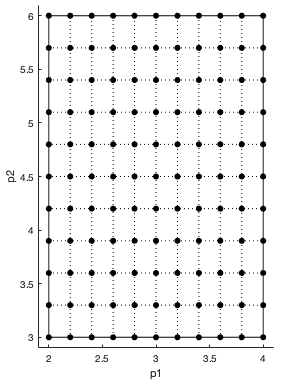For details, see Elementary Effects for Global Sensitivity Analysis.

Data Types: `double`

Step size for computing elementary effects, specified as a positive integer between 1 and `GridLevel`. The step size is measured in terms of grid points between neighboring points. The following figure shows examples of different grid delta values.For details, see Elementary Effects for Global Sensitivity Analysis.

Data Types: `double`

Flag to use the absolute values of elementary effects, specified as `true` or `false`. By default, the function uses the absolute values of elementary effects. Using nonabsolute values can average out when calculating the mean. For details, see Elementary Effects for Global Sensitivity Analysis.

Data Types: `logical`

Method to generate parameter samples, specified as one of the following:

• `"lhs"` — Use low-discrepancy Latin hypercube samples.

• `"random"` — Use uniformly distributed random samples.

The function selects generated parameter samples by sampling the grid points.

Simulation stop time, specified as a nonnegative scalar. If you specify neither `StopTime` nor `OutputTimes`, the function uses the stop time from the active configuration set of the model. You cannot specify both `StopTime` and `OutputTimes`.

Data Types: `double`

Simulation output times, specified as a numeric vector. The function computes the elementary effects at these output time points. You cannot specify both `StopTime` and `OutputTimes`. By default, the function uses the reported time points of the first model simulation.

Example: `[0 1 2 3.5 4 5 5.5]`

Data Types: `double`

Flag to run model simulations in parallel, specified as `true` or `false`. When the value is `true` and Parallel Computing Toolbox™ is available, the function runs simulations in parallel.

Data Types: `logical`

Flag to turn on model acceleration, specified as `true` or `false`.

Data Types: `logical`

Method for interpolation of model responses to a common set of output times, specified as a character vector or string. The valid options follow.

• `"interp1q"` — Use the `interp1q` function.

• Use the `interp1` function by specifying one of the following methods:

• `"nearest"`

• `"linear"`

• `"spline"`

• `"pchip"`

• `"v5cubic"`

• `"zoh"` — Specify zero-order hold.

Data Types: `char` | `string`

Flag to show the progress of model simulations by displaying a wait bar, specified as `true` or `false`. By default, no wait bar is displayed.

Data Types: `logical`

## Output Arguments

collapse all

Results containing means and standard deviations of elementary effects, returned as a `SimBiology.gsa.ElementaryEffects` object. The object includes information such as the mean and standard deviation of elementary effects as well as parameter samples and model simulations used to compute the elementary effects.

collapse all

### Elementary Effects for Global Sensitivity Analysis

`sbioelementaryeffects` lets you assess global sensitivity of a model response with respect to variations in model parameters.

Consider a simple case with one sensitivity input parameter P. The elementary effect EE of P with respect to a model response R is defined as follows.

$E{E}_{P}\left(x\right)=\frac{R\left(x\right)-R\left(x+delta\right)}{delta}$

Here, EEP(x) is the elementary effect of P . R(x) and R(x+delta) are model responses at a specific time or the values of observables, evaluated for parameter values `x` and `x+delta`.

In the general case of k sensitivity input parameters, x is a vector of different parameter values, ```x = [v1,v2,v3,…,vk]```. The elementary effect of the ith parameter is computed as follows.

$\begin{array}{c}E{E}_{Pi}\left(x\right)=\frac{R\left({v}_{1},{v}_{2},{v}_{3},...,{v}_{i},...,{v}_{k}\right)-R\left({v}_{1},{v}_{2},{v}_{3},...,{v}_{i}+delt{a}_{i},...,{v}_{k}\right)}{delt{a}_{i}}\\ =\frac{R\left(x\right)-R\left(x+{e}_{i}×delt{a}_{i}\right)}{delt{a}_{i}}\end{array}$

Here, ei is the ith canonical unit vector. Thus, calculating the elementary effects of all parameters `P1,P2,P3,…,Pk` requires `k+1` model simulations.

The function provides two methods (`'PointSelection'`) to select a set of `k+1` points required to compute these elementary effects.

• `radial` — This method  uses k points, $x±{e}_{1}×delt{a}_{1},x±{e}_{2}×delt{a}_{2},...,x±{e}_{k}×delt{a}_{k}$, that are arranged around one center point x to compute elementary effects for each of k parameters.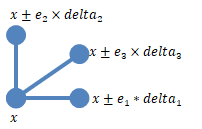• `chain` — This method  uses chains of points, instead of radially-arranged points around a center points:

$x+\sum _{i=1}^{n}{e}_{i}×delt{a}_{i},\text{where}\text{​}\text{\hspace{0.17em}}n=0,1,...,k$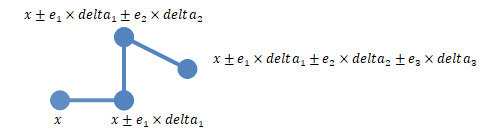To get the mean and standard deviation of elementary effects, the function computes N (`'NumberSamples'`) elementary effects per parameter, which requires `N*(k+1)` simulations. By default, the function reports the mean and standard deviation of absolute elementary effects of each parameter `P1,P2,P3,…,Pk`.

• The mean of elementary effects explains whether variations in parameter P have any effect on response R on average.

• The standard deviation explains whether the sensitivity change is dependent on the location in the parameter domain.

The function uses the absolute elementary effects by default because the elementary effects can average out when calculating the mean otherwise. Optionally, you can set the `'AbsoluteEffects'` name-value argument to `false` to get the means and standard deviations of nonabsolute elementary effects.

The function reports the points used to compute elementary effects in the `ParameterSamples` property of the returned results object. Each block of `k+1` rows in the table of `ParameterSamples` corresponds to the k+1 radial or chained points used to compute the elementary effects. The `SimulationInfo.SimData` property of the results object contains the corresponding model simulations. The function samples the points from the parameter grid defined by `'GridLevel'` and `'GridDelta'`. The following figure illustrates a simple case with two sensitivity inputs (y1 and y2) with `'NumberSamples'` = 2 using the `chain` `'PointSelection'` method.Morris, Max D. “Factorial Sampling Plans for Preliminary Computational Experiments.” Technometrics 33, no. 2 (May 1991): 161–74.

 Sohier, Henri, Jean-Loup Farges, and Helene Piet-Lahanier. “Improvement of the Representativity of the Morris Method for Air-Launch-to-Orbit Separation.” IFAC Proceedings Volumes 47, no. 3 (2014): 7954–59.